# Complexity Explorer Santa Few Institute## Introduction to Information Theory

• Measuring Information: Bits
• Using Bits to Count and Label
• Physical Forms of Information
• Fundamental Formula of Information
• Computation and Logic: Information Processing
• Mutual Information
• Communication Capacity in a Noisy Channel
• Shannon's Coding Theorem
• Homework Solutions

#### 3.1 Information and Probability » Quiz Solutions

Question 1:

Since the coin is fair, the expected number of tails among a million tosses is 0.5 * 1,000,000 = 500,000. Note that this is also the expected number of heads.

Question 2:

Recalling the formula given in the video for the actual number of tails,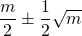, where m is the expected number of tails (the same formula applies for heads too).

In the first part of the formula we find that by substituting the number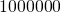for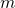the expected number of  tails is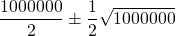which is equal to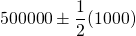which is equal to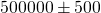.

Thus, the difference between the actual and the expected number of tails will be approximately 500.